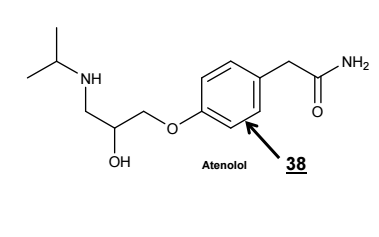# Problem: The number of chemical bonds between two atoms is described by the  bond order. For example, a single bond has a bond order of 1, and a triple bond has a bond order of 3. In a resonance hybrid, the bond order need not be an integer quantity! Look at the carbon-carbon bond labeled  38 in the structure of atenolol above. What is the  bond order of this carbon-carbon bond? Pick the  one choice below which best answers this question. A. 0.5 B. 1.0 C. 1.5 D. 2.0 E. 2.5 F. 3.0 G. None of these

###### Problem Details

The number of chemical bonds between two atoms is described by the  bond order. For example, a single bond has a bond order of 1, and a triple bond has a bond order of 3. In a resonance hybrid, the bond order need not be an integer quantity! Look at the carbon-carbon bond labeled  38 in the structure of atenolol above. What is the  bond order of this carbon-carbon bond? Pick the  one choice below which best answers this question.

A. 0.5

B. 1.0

C. 1.5

D. 2.0

E. 2.5

F. 3.0

G. None of these